Next: Gauge Transformations in Electromagnetism Up: Quantum Dynamics Previous: Schrödinger Wave Equation

# Charged Particle Motion in Electromagnetic Fields

The classical Hamiltonian for a particle of mass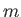and charge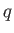moving under the influence of electromagnetic fields is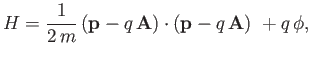(3.71)

where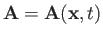and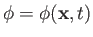are the vector and scalar potentials, respectively . These potentials are related to the familiar electric and magnetic field-strengths,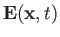and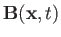, respectively, via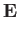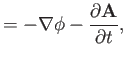(3.72)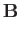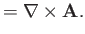(3.73)

Let us assume that expression (3.71) is also the correct quantum mechanical Hamiltonian for a charged particle moving in electromagnetic fields. Obviously, in quantum mechanics, we must treat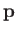,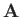, and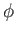as operators that do not necessarily commute.

The Heisenberg equations of motion for the components of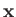are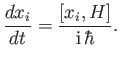(3.74)

However,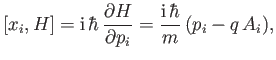(3.75)

where use has been made of Equations (3.33) and (3.71). It follows that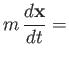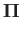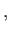(3.76)

where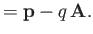(3.77)

Here,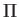is referred to as the mechanical momentum, whereasis termed the canonical momentum.

It is easily seen that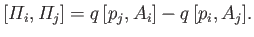(3.78)

However,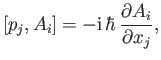(3.79)

where we have employed Equation (3.34). Thus, we obtain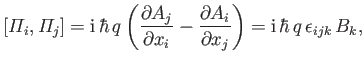(3.80)

because [from Equation (3.73)]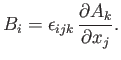(3.81)

Here,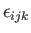is the totally antisymmetric tensor (that is,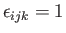if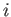,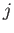,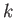is a cyclic permutation of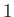,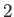,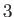;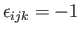if,,is an anti-cyclic permutation of,,; and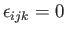otherwise), and we have used the standard result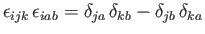, as well as the Einstein summation convention (that repeated indices are implicitly summed fromto) .

We can write the Hamiltonian (3.71) in the form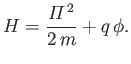(3.82)

The Heisenberg equation of motions for the components ofare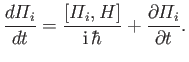(3.83)

Here, we have taken into account the fact that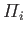depends explicitly on time through its dependence on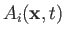. However,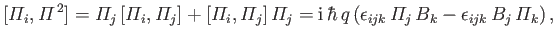(3.84)

where use has been made of Equation (3.80). Moreover,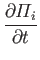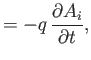(3.85)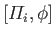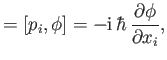(3.86)

where we have employed Equation (3.33). The previous five equations yield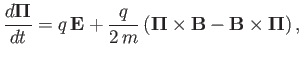(3.87)

which can be combined with Equation (3.76) to give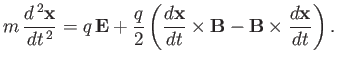(3.88)

This equation of motion is a generalization of the Ehrenfest theorem that takes electromagnetic fields into account. The fact that Equation (3.88) is analogous in form to the corresponding classical equation of motion (given that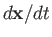and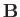commute in classical mechanics) justifies our earlier assumption that Equation (3.71) is the correct quantum mechanical Hamiltonian for a charged particle moving in electromagnetic fields.Next: Gauge Transformations in Electromagnetism Up: Quantum Dynamics Previous: Schrödinger Wave Equation
Richard Fitzpatrick 2016-01-22